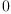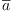login  home  contents  what's new  discussion  bug reports help  links  subscribe  changes  refresh  edit

Why this evalutate to zero ?

fricas
A: Complex Polynomial Integer
Type: Void
fricas
A*conjugate A - A^2(1)
Type: Complex(Polynomial(Integer))

Definitely wrong. It looks like the source of the problem is:

fricas
conjugate(a)(2)
Type: Expression(Integer)

Bill Page wrote:

Of course I agree that it is wrong. But what would you expect the answer to:

  conjugate(a)


to be? It could return unevaluated as just:

  conjugate(a)


but then what should the Type: be? Is such an expression still necessarily a Complex Polynomial Integer?

This is dangerously close to the discussion that we had some months ago about the meaning of "indeterminants", objects which have a type but no specific value. For example, I can say:

  A:Integer


but if I write:

  A+1


If you answered "yes" to the idea that conjugate(a) is still of type Complex Polynomial Integer, then surely A+1 is still of type Integer is the same sense, but Axiom complains that "A has not been given a value".

Perhaps evaluating the expression:

  conjugate(a)


should also complain about the lack of a value?

complex numbers --root, Wed, 29 Dec 2004 00:47:32 -0600 reply
seems to be a categorical error of some sort.

A: Complex Polynomial Integer

tells the system that A is expected to have a value which is Complex Polynomial Integer.

conjugate works on values, not potential values.

Thus, conjugate(A) has no meaning as A has no value. This should probably be an error.

If axiom could work with so that conjugate worked on the type then axiom could work at some sort of an axiomatic level rather than a symbolic computation level. Perhaps when we join forces with the ACL2 crowd we could state certain theorems and have them applied in the absence of a value.

Tim

Tim,

I know that this is opening up the whole big subject again, but I do think that Axiom is already "two-faced" about this.

Consider for example that we can write:

(7) -> A: Complex Polynomial Integer Type: Void (8) -> B: Complex Polynomial Integer Type: Void (9) -> A+B

(9) B + A Type: Complex Polynomial Integer

Neither A or B "has a value" but Axiom has no trouble agreeing that A+B is still of type Complex Polynomial Integer.

I do not see any essential difference between this and

(10) -> A:Integer Type: Void (11) -> B:Integer Type: Void (12) -> A+B

A is declared as being in Integer but has not been given a value.

Regards, Bill Page.

complex numbers --root, Wed, 29 Dec 2004 01:36:08 -0600 reply
Clearly you're right and I agree with you.

The problem, as I see it, is that there are subtle degrees of meaning that are easily stepped around when you work on paper but must be clarified in computational mathematics.

A: Integer

might mean that 1) A will hold a value which is an integer under interpretation (1)

A+1

is an error since A has no value. But what does the type of an unbound thing mean? Lisp assigns types to the values, not the boxes. This is like labeling a box television.

or perhaps that 2) A is an indefinite object of type integer under interpretation (2)

A+1

is another indeterminant integer, where + comes from Integer.

or perhaps that 3) A obeys the laws applied to integers under interpretation (3)

A+1

is a polynomial with 2 integers, representing a constant, where + comes from Polynomial.

or perhaps that 4) A is a symbol which hold integers under interpretation (4)

A+1

is a polynomial in A with integer coefficients ignoring 'A's type where + comes from Polynomial.

or perhaps that 5) A is an element of a Ring and theorems can be applied under interpretation (5)

A+1

is B, a new member of the Ring since + is a ring operator and both A and 1 are ring elements. Ideally Axiom's types would be decorated with axioms, like the ring axioms making reasoning about unbound but typed objects possible. The + comes from the Category axioms of Integer.

The exact interpretation chosen appears to be dictated by the underlying code and is not the same everywhere.

Axiom is the product of many people, some of whom have chosen different interpretations. Indeed, some of the interpretations didn't exist before the computational aspects of mathematics came into play.

There are quite a few areas of research that could be followed. Indeed, working out the implications of the several meanings of A is a PhD? topic, and a rather hard one at that. The issue heads off into questions of provisos, questions of reasoning with theorems and axioms, etc.

Axiom's main strength has always been as a research platform where it is possible to work out these ideas and reduce them to practice.

Unfortunately, research funding seems nowhere to be found.

Tim

complex numbers --Bob McElrath?, Wed, 29 Dec 2004 12:12:44 -0600 reply
root [daly@idsi.net]? wrote:
Clearly you're right and I agree with you.

The problem, as I see it, is that there are subtle degrees of meaning that are easily stepped around when you work on paper but must be clarified in computational mathematics.

Why cannot we allow all? I expect I should be able to coerce A+1 to an Integer, Polynomial Integer, Expression Integer, etc. The default seems to be Integer, which seems fine.

## The error seems to be in the Polynomial domain:

(16) -> c:Complex Integer Type: Void (17) -> conjugate(c)

c is declared as being in Complex Integer but has not been given a value. (17) -> c:Complex Polynomial Integer Type: Void (18) -> conjugate(c)

(18) c Type: Complex Polynomial Integer

## Adding the Polynomial to its domain causes this to go wrong...

(19) -> c:Polynomial Complex Integer Type: Void (20) -> conjugate(c) There are 4 exposed and 1 unexposed library operations named conjugate having 1 argument(s) but none was determined to be ...

I'm surprised this doesn't work. A Polynomial on a Ring is still a member of that ring and should inherit its functions. (in this case, conjugate) It also strikes me that Polynomial Complex Integer is the proper type here, not Complex Polynomial Integer...clearly they are inequivalent.

A: Integer

might mean that 1) A will hold a value which is an integer under interpretation (1)

A+1

is an error since A has no value. But what does the type of an unbound thing mean? Lisp assigns types to the values, not the boxes. This is like labeling a box television.

This is the approach taken by Integer, Complex, and Float it seems.

or perhaps that 2) A is an indefinite object of type integer under interpretation (2)

A+1

is another indeterminant integer, where + comes from Integer.

or perhaps that 3) A obeys the laws applied to integers under interpretation (3)

A+1

is a polynomial with 2 integers, representing a constant, where + comes from Polynomial.

I cannot seem to construct an example of type (3). Given: c:Integer and I want to construct a Polynomial Integer containing c, how would I do it?

or perhaps that 4) A is a symbol which hold integers under interpretation (4)

A+1

is a polynomial in A with integer coefficients ignoring 'A's type where + comes from Polynomial.

The type of A cannot be ignored since A+1 must Polynomial must have a Field such as Integer in its constructor.

or perhaps that 5) A is an element of a Ring and theorems can be applied under interpretation (5)

A+1

is B, a new member of the Ring since + is a ring operator and both A and 1 are ring elements. Ideally Axiom's types would be decorated with axioms, like the ring axioms making reasoning about unbound but typed objects possible. The + comes from the Category axioms of Integer.

Now this truly is a research project. ;)

The exact interpretation chosen appears to be dictated by the underlying code and is not the same everywhere.

Axiom is the product of many people, some of whom have chosen different interpretations. Indeed, some of the interpretations didn't exist before the computational aspects of mathematics came into play.

I think this must be clarified, and a unifying set of assumptions be applied across all domains.

There are quite a few areas of research that could be followed. Indeed, working out the implications of the several meanings of A is a PhD? topic, and a rather hard one at that. The issue heads off into questions of provisos, questions of reasoning with theorems and axioms, etc.

This does not seem so hard, but maybe I am being naive.

Cheers, Bob McElrath? [Univ. of California at Davis, Department of Physics]?

Complex domain constructor --Bill Page, Thu, 18 Mar 2010 14:09:05 -0700 reply
[Complex]? is domain constructor which takes a CommutativeRing? as an argument.

Complex builtin? --Bill Page, Thu, 18 Mar 2010 14:15:08 -0700 reply
Some properties of the [Complex]? domain seem to be built-in to the interpreter. For example Martin Baker wrote:
  Date: Thu, Mar 18, 2010 at 1:53 PM
Subject: [fricas-devel] Hypercomplex numbers


How would one go about building a set of domains to represent hypercomplex numbers without defining each one individually as a separate domain? For example: complex numbers, double numbers, dual numbers, dual complex, dual quaternion, dual vectors, split complex, split quaternion and so on.

What I had in mind was 3 domains which all have one real dimension and a second dimension which squares as follows:

  Complex: i^2 = -1
Double D^2 = +1
Dual e^2 =0


Then these types could be combined in various combinations, for example:

  myType:Complex Complex Dual Integer


down to any depth to produce the required hypercomplex type (even if the algebra gets degraded).

The problem is that complex numbers are defined over CommutativeRing? and even types like this are not valid.

fricas
myType:Complex Complex Integer
Complex(Complex(Integer)) is not a valid type.

Even if this was accepted as a valid type we would need to be able to define how the product of the two algebras would be calculated, not necessarily direct product but semi-direct product or Cayley-Dickson doubling process.

There would also be practical issues like if the outer Complex uses %i then the inner Complex would need to use %j and so on. I realise that in this case we could just use quaternion but I am interested what the general solution would be.

There are other cases like this, for instance Clifford algebras over Clifford algebras?

Would I be right in assuming that FriCAS can't do this sort of thing at the moment?

Martin

 Subject:   Be Bold !! ( 14 subscribers )